Courses
Courses for Kids
Free study material
Offline Centres
MoreLast updated date: 08th Dec 2023
Total views: 594.6k
Views today: 15.94k

## Revision Notes for CBSE Class 8 Maths Chapter 3 - Free PDF Download

Revision Notes for CBSE Class 8 Mathematics Chapter 3 Understanding Quadrilaterals are quite useful for swiftly reviewing the whole chapter on exam days. In the form of a summary, the revision notes encompass all essential formulae and concepts presented in the chapter. Students do not need to be concerned about the large chapters to be covered during test time; simply refer to the revision notes and they are finished.

Well qualified faculties at Vedantu have designed CBSE Class 8 Maths Notes for Chapter 3 Understanding Quadrilaterals in such a way that the whole chapter is covered in a short summary. Vedantu’s revision notes cover all the important concepts and formulas related to quadrilaterals. Students can no doubt depend upon these revision notes while revising the chapters during the exam time.  If you wish to have an overview of a chapter at a glance, Vedantu revision notes will do this for you. Vedantu is a platform that provides free NCERT Solution and other study materials for students. Download Class 8 Maths NCERT Solutions and NCERT Solutions for Class 8 Science to help you to revise complete syllabus ans score more marks in your examinations.

## Important Topics Covered in CBSE Class 8 Maths Chapter 3 Understanding Quadrilaterals

The below list contains the important topics of the CBSE Class 8 Maths Chapter 3, which have appeared at least once in previous years’ examinations. So, students must go through these thoroughly while preparing for the upcoming exams.

1. Introduction to Plane Surfaces

2. Polygons

3. Classification of Polygons

4. Diagonals

5. Convex and Concave Polygons

6. Regular and Irregular Polygons

7. Angle Sum Property in Quadrilaterals

8. Sum of the Measures of the Exterior Angles of a Polygon

• Trapezium

• Kite

• Parallelogram

•  Elements of a Parallelogram

•  Angles of a Parallelogram

• Diagonals of a Parallelogram

1. Some Special Parallelograms

• Rhombus

• Rectangle

• Square

Also, check CBSE Class 8 Maths revision notes for All chapters:

## Access Class 8 Maths Chapter 3 - Understanding Quadrilaterals Notes in 30 Minutes

Parallelogram:

A quadrilateral with each pair of opposite sides parallel.

1. Opposite sides are equal.

2. Opposite angles are equal.

3. Diagonals bisect one another.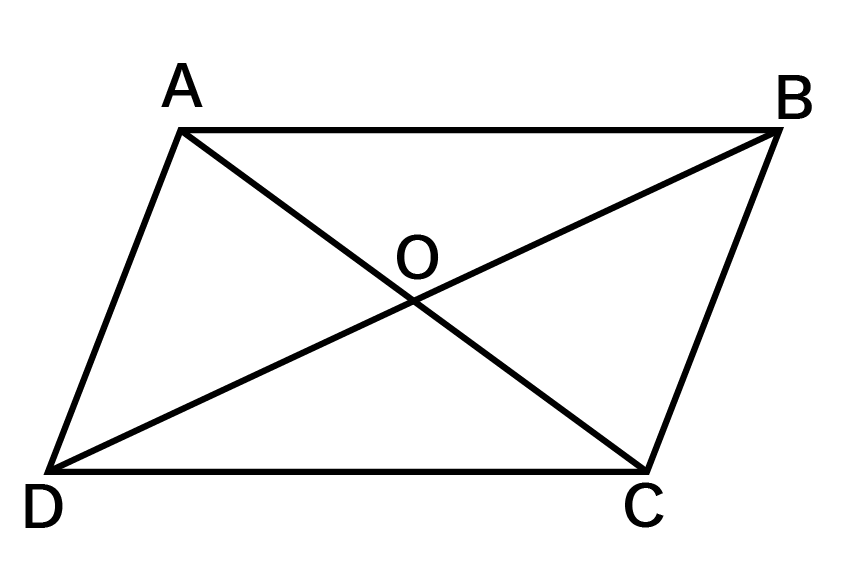Rhombus:

A parallelogram with sides of equal length.

1. All the properties of a parallelogram.

2. Diagonals are perpendicular to each other.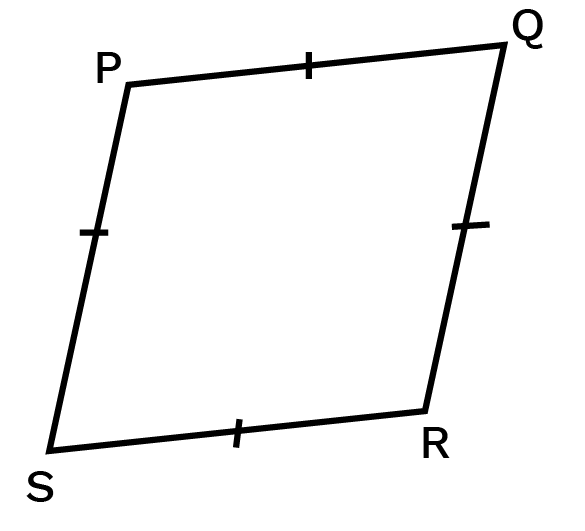Rectangle:

A parallelogram with a right angle.

1. All the properties of a parallelogram.

2. Each of the angles is a right angle.

3. Diagonals are equal.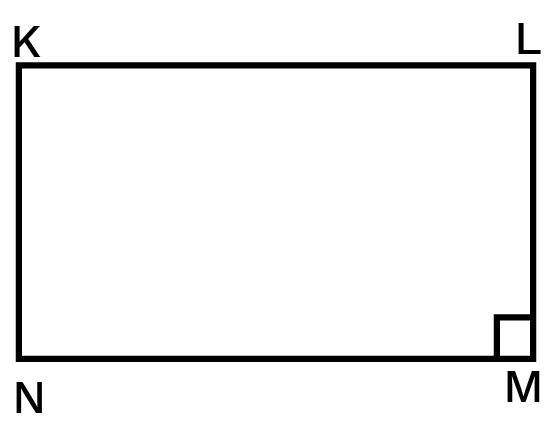Square:

A rectangle with sides of equal length.

All the properties of a parallelogram, rhombus, and a rectangle.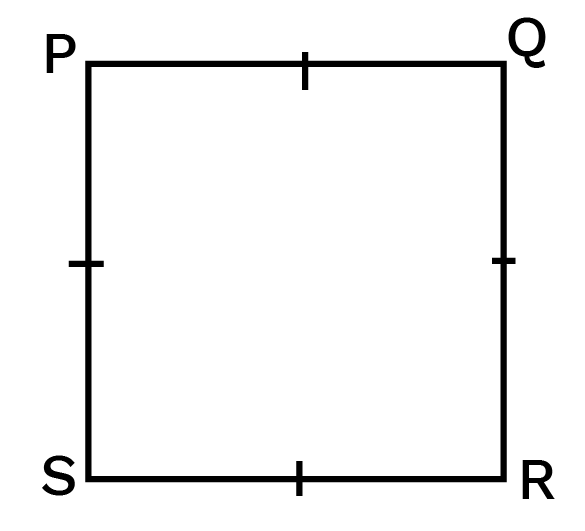Kite:

A quadrilateral with exactly two pairs of equal consecutive sides.

1. The diagonals are perpendicular to one another.

2. One of the diagonals bisects the other.

3. From figure,

\$m\angle B=m\angle D\$ but \$m\angle A\ne m\angle C\$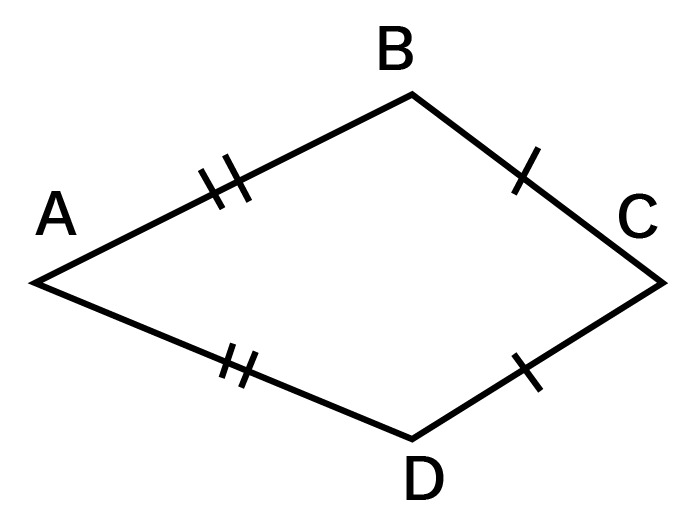Trapezium:

A quadrilateral having exactly one pair of parallel sides.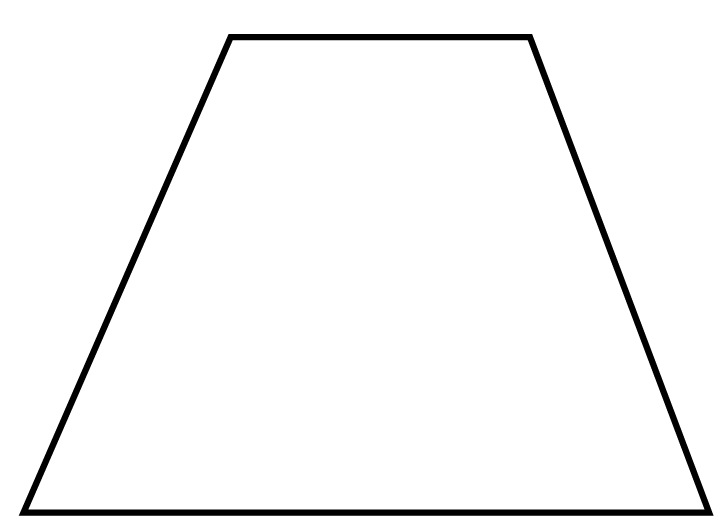Diagonal:

A simple closed curve is made up of only line segments.

A line segment connecting two non-consecutive vertices of a polygon is called diagonal.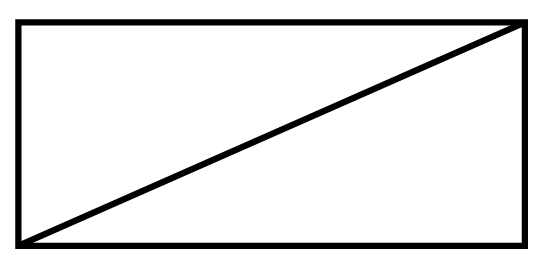Convex:

The measure of each angle is less than \${{180}^{0}}\$.

Concave:

The measure of at least one angle is more than \${{180}^{0}}\$.

A polygon having four sides.

(i) Sides:

Line segments joining the points.

(ii) Vertices:

Point of intersection of two consecutive sides.

(iii) Opposite sides:

Two sides of a quadrilateral having no common endpoint.

(iv) Opposite Angles:

Two angles of a quadrilateral not having a common arm.

(v) Diagonals:

A line segment is obtained by joining the opposite vertices.

Two angles of a quadrilateral having a common arm.

Two sides of a quadrilateral having a common endpoint.

Continuous revision is one of the most effective techniques for students to overcome their dread of taking final examinations. The CBSE Mathematics Chapter 3 Class 8 Notes from Vedantu include content created by our finest teachers who have taught in the CBSE board. Students will be able to improve their Class 8 test scores by using these review notes. The notes have been made to be simple to grasp. Vedantu's revision notes are available in PDF format for simple download. Students may use the Understanding Quadrilaterals Class 8 Notes PDF to review the whole curriculum and improve their test scores.

Students feel the pressure of taking their final exams. To vanish this pressure, they must revise thoroughly and practice well. By referring to Vedantu’s Class 8 revision notes, students can not only score well, but they can also understand concepts better and enjoy the process of learning instead of cramming last minute. The notes are all easy to understand because they are very simple and to the point.

A figure bounded by four line segments such that no three of them are parallel form a quadrilateral.

A quadrilateral has four sides, four vertices, and four angles.

Thus below figure ABCD is a quadrilateral that is bounded by four sides i.e AB, BC, CD, and AD. The four vertices are A, B, C, and D. ∠A, ∠B, ∠C, and ∠D make the four angles of the quadrilateral. And it is written as □ABCD and read as quadrilateral ABCD.

A line segment drawn from one vertex to the opposite vertex is called the diagonal of the quadrilateral. In the below figure, segment AC and BD are the diagonals of the quadrilateral ABCD.

Opposite SIdes: Two sides of a quadrilateral are opposite sides if the sides have no common vertex. In the above figure side AB and DC; Side AD and BC are the two pairs of opposite sides.

Opposite Angles: Two angles of a quadrilateral are opposite angles if they don’t have any common arm. In the above figure, ∠A and  ∠C;  ∠B and ∠D, are two pairs of opposite angles.

Adjacent Sides: Two Sides of a quadrilateral are said to be adjacent if the sides have a common vertex. In the above figure, Side AB and BC; Side BC and CD; Side CD and DA; Side DA and AB are the four pairs of adjacent sides and are also called consecutive sides.

Adjacent Angles: Two angles of a quadrilateral is said to be adjacent angles if the angles have a common side or an arm. In the above figure  ∠A and  ∠B;  ∠B and ∠C, ∠C and  ∠D;  ∠D and ∠A are the four pairs of adjacent angles also called consecutive angles.

There are basically six types of quadrilaterals. They are as follows,

Parallelogram: A quadrilateral that has its opposite sides congruent and parallel to each other is a parallelogram. Its opposite angles are also congruent to each other.Rectangle: A quadrilateral that has its opposite sides equal and all the angles are at right angles(900) is called a rectangle.Square: A quadrilateral that has all its four sides equal and opposite sides are parallel, and all the angles at right angles(900), is called a square.Rhombus: A quadrilateral has all its sides equal and its diagonals bisect each other at 900 is called a rhombus.Trapezium: A quadrilateral that has only one pair of sides are parallel and the two sides are non-parallel is called a trapezium. The sides may not be equal to each other.Kite: A quadrilateral that has two pairs of equal adjacent sides and unequal opposite sides is Kite.As we know that a quadrilateral has four angles. The sum of the angles of the quadrilateral is 3600.

The sum of all the angles of the □ABCD ∠A +∠B + ∠C + ∠D = 360°.

In the case of square and rectangle, the measure of all the angles is 900.

Therefore we have ∠A = ∠B = ∠C = ∠D = 90°.

### Angle Sum Property of Quadrilateral Theorem

The sum of the measures of four angles of a quadrilateral is 3600

i.e ∠ABC + ∠BCD + ∠CDA + ∠DAB = 360°.

### Benefits of Understanding Quadrilaterals Class 8 Notes By Vedantu

Understanding Quadrilaterals Class 8 Notes prepared by our experts at Vedantu contain formulae, definitions, diagrams, and quick explanations of the important concepts which make it easier for the school students to grasp the topics easily.

The highlighted notes, easy language and appropriate reference images help students to understand them and remember them easily. Since these notes are prepared by subject experts at Vedantu they give students an edge over their peers when it comes to understanding the theory as well as applications of a concept.

If you haven’t yet downloaded the CBSE Class 8 Maths Revision Notes for Chapter 3 Understanding Quadrilaterals yet, then you’re losing out on the opportunity of studying from the free downloadable expert-curated accurate study material. Further, if you download the free PDF of these Maths revision notes and study them diligently, you will be able to steer your preparation in the right direction with boosted confidence.

## Conclusion

In conclusion, the Class 8 Revision Notes for CBSE Maths Chapter 3 - Understanding Quadrilaterals provide a valuable resource for students to revise and reinforce their understanding of the topic. These revision notes, available as a free PDF download, cover the important concepts, properties, and characteristics of quadrilaterals in a concise and organized manner. The revision notes begin by introducing the definition of a quadrilateral and its various types, such as parallelograms, rectangles, squares, rhombuses, and trapeziums. Each type is explained with clear explanations and examples, allowing students to grasp the distinguishing features of each quadrilateral.

## FAQs on Class 8 Revision Notes for CBSE Maths Chapter 3 Understanding Quadrilaterals (Free PDF Download)

1. When Should You Refer to The Notes?

You should study these revision notes by Vedantu:

• Immediately After you Finish a Chapter - Quickly capture the important points, definitions, formulae, theorems and diagrams covered in the chapter and cement them in your mind.

• During your Weekly Revisions - Check if you have already forgotten an important point, theorem or formula and revise it once again.

• Just before Tests and Exams - To cover the chapter quickly at a glance without spending too much time on the content that is less important.

2. What are the topics covered in Class 8 Chapter 3 Understanding Quadrilaterals?

The topics covered in Class 8 Chapter 3 Understanding Quadrilaterals are Polygons, Classification of Polygons, Diagonals, Convex and Concave Polygons, Regular and Irregular Polygons, Angle Sum Property, Sum of the measures of the Exterior Angles of Polygon, Different kinds of Quadrilateral, Trapezium, Kite, Parallelogram, Elements of a parallelogram, Angles of a parallelogram, Diagonals of a parallelogram, Some Special Parallelograms, Rhombus, A rectangle, and A square.

3. How to gain in-depth knowledge of Chapter 3 of Class 8 Maths?

The CBSE NCERT questions are answered in the NCERT Solutions for Chapter 3 of Class 8 Maths. The exercise questions are answered in an ideal manner and they best suit the questions from an exam point of view. All questions are prepared by subject matter experts with decades of experience. All these important questions are based on the latest CBSE guidelines, making scheme and syllabus. Referring to these questions will help students get an in-depth understanding of Chapter 3.

4. How to score maximum marks in Chapter 3 of Class 8 Maths examination?

One of the most effective strategies for nervous students to overcome their anxiety about writing final examinations is to revise frequently. They must also practice NCERT’s solved examples and exercises regularly. Mock question papers and questions from different reference books also provide an edge to students and prepares them on a cumulative basis. Vedantu's CBSE Maths Chapter 3 Class 8 Notes contain content provided by our top instructors, all of whom have taught on the CBSE board. Students will be able to get better grades in their Class 8 examinations if they use these revision notes. Understanding Quadrilaterals Class 8 Notes PDF may be downloaded by students to help them review the whole curriculum and improve their test scores. The notes and solutions are free of cost and also available on Vedantu’s website(vedantu.com) and mobile app.

5. What are the benefits of revision notes for Chapter 3 of Class 8 Maths?

Class 8 Understanding Quadrilaterals Formulae, definitions, illustrations, and brief explanations of key ideas are included in the notes created by our specialists at Vedantu, making it easier for school children to comprehend the concepts. Students may readily comprehend and recall the notes because of the highlighted points, simple language, and relevant reference pictures. Because these notes are written by Vedantu topic specialists, students will have an advantage over their classmates when it comes to grasping the theory and applications of a concept.

6. What are the different types of quadrilaterals?

Quadrilaterals are divided into six categories. A parallelogram is a quadrilateral with opposing sides that are congruent and parallel to each other. Its opposing angles are likewise in line with one another. A rectangle is a quadrilateral with opposing equal sides and all angles at right angles (90 degrees). A square is a quadrilateral with all four sides being equal, opposing sides being parallel, and all angles being right angles (90 degrees). A rhombus is a quadrilateral with all of its sides equal and diagonals that bisect each other at 90 degrees. A trapezium is a quadrilateral with only one set of parallel sides and two sides that are not parallel and may or may not be equal. A kite is a quadrilateral with unequal opposed sides and two sets of equal adjacent sides.

7. What are the elements of a quadrilateral?

There are numerous distinct components in a quadrilateral. The line segments that link the vertices are known as sides. Vertices are the intersections of two adjacent sides. A quadrilateral's two opposing sides with no shared termination are referred to as opposite sides. The two quadrilateral angles with no common arm are known as opposite angles. Diagonals are produced by joining the vertices on opposing sides of a line segment. Adjacent Angles are two quadrilateral angles that share a common arm. Adjacent Sides are a pair of quadrilateral sides that share a common termination.